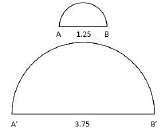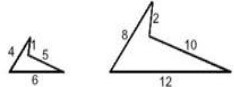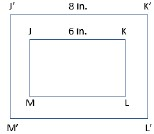# MA.8.GR.2.2Export Print
Given a preimage and image generated by a single dilation, identify the scale factor that describes the relationship.

### Clarifications

Clarification 1: Instruction includes the connection to scale drawings and proportions.

Clarification 2: Instruction focuses on the preservation of similarity and the lack of preservation of congruence when a figure maps onto a scaled copy of itself, unless the scaling factor is 1.

General Information
Subject Area: Mathematics (B.E.S.T.)
Strand: Geometric Reasoning
Status: State Board Approved

## Benchmark Instructional Guide

• Dilation
• Scale Factor

### Vertical Alignment

Previous Benchmarks

Next Benchmarks

### Purpose and Instructional Strategies

In grade 7, students solved mathematical and real-world problems involving scale factors. In grade 8, students determine the scale factor that describes the relationship after a single dilation. In Geometry, students will use dilations to study similarity.
• Instruction includes the use of real-world examples that do not have to be a geometric figure.
• For example, projections, photocopies and maps have images generated by a single dilation.
• Students will need to understand scale factor to help in the idea of an image enlarging or reducing. A scale factor between 0 and 1 will be a reduction in the image. A scale factor that is greater than one will result in an enlargement of the image.
• Transformations can be noted using the prime notation (′) for the image and its vertices. The preimage and its vertices will not have prime notation.
• For example, the picture below showcases a single dilation.• The expectation of this benchmark is not to represent a dilation on the coordinate plane as this will be included in MA.8.GR.2.3 instruction.

### Common Misconceptions or Errors

• Students may incorrectly visualize the images with scale factors. To address this misconception, include practice in visualizing the reduction and enlargement based on the scale factors.

### Strategies to Support Tiered Instruction

• Instruction includes practice in visualizing the reduction and enlargement based on the scale factors for students that incorrectly visualize images with scale factors.
• For example, two figures that have the same shape are said to be similar. When two figures are similar, the ratios of the lengths of their corresponding sides are equal and corresponding angles are congruent. Similar figures have the same shape, but not necessarily the same size.• Teachers can help students understand that two figures that have the same shape are said to be similar. When two figures are similar, the ratios of the lengths of their corresponding sides are equal. In example 1, the corresponding sides are 8:4, 2:1, 10:5, and 12:6. These ratios are equal to 2, meaning the shapes must be similar. The figures do not have to be the same size in order to be similar.
• Instruction include practice in visualizing the reduction and enlargement based on the scale factors.

The height of a document on your computer is 20 centimeters. When you change the setting to zoom in or out, you changed it from 100% to 25%. The new image of your document is a dilation of your original document, the preimage. Determine the scale factor and the height of the new image.

### Instructional Items

Instructional Item 1
Does the image show reduction or enlargement from the quadrilateral $J$$K$$L$$M$? What is the scale factor?*The strategies, tasks and items included in the B1G-M are examples and should not be considered comprehensive.

## Related Courses

This benchmark is part of these courses.
1205050: M/J Accelerated Mathematics Grade 7 (Specifically in versions: 2014 - 2015, 2015 - 2020, 2020 - 2022, 2022 and beyond (current))
1205070: M/J Grade 8 Pre-Algebra (Specifically in versions: 2014 - 2015, 2015 - 2022, 2022 and beyond (current))
1204000: M/J Foundational Skills in Mathematics 6-8 (Specifically in versions: 2014 - 2015, 2015 - 2022, 2022 and beyond (current))
7812030: Access M/J Grade 8 Pre-Algebra (Specifically in versions: 2014 - 2015, 2015 - 2018, 2018 - 2019, 2019 - 2022, 2022 and beyond (current))

## Related Access Points

Alternate version of this benchmark for students with significant cognitive disabilities.
MA.8.GR.2.AP.2: Given a preimage and image describe the effect the dilation has on the two figures.

## Related Resources

Vetted resources educators can use to teach the concepts and skills in this benchmark.

## Lesson Plan

How does scale factor affect the areas and perimeters of similar figures?:

In this lesson plan, students will observe and record the linear dimensions of similar figures, and then discover how the values of area and perimeter are related to the ratio of the linear dimensions of the figures.

Type: Lesson Plan

## Perspectives Video: Professional/Enthusiast

All Circles Are Similar- Especially Circular Pizza!:

What better way to demonstrate that all circles are similar then to use pizzas! Gaines Street Pies explains how all pizza pies are similar through transformations.